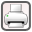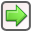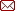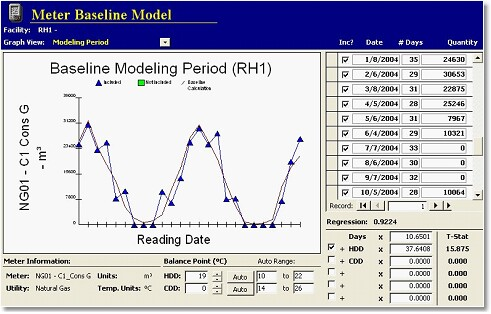﻿ Report Structure > Utility Invoice Analysis
 Navigation:  Report Structure > Utility Invoice Analysisrev. 2011-07-04Utility invoice analysis is a mathematical processing of recent utility billing history to illustrate how energy is presently being used at the site, how that usage compares to other similar facilities, and the general magnitude of energy conservation opportunities.

Utility invoice analysis is quite involved, so only the summary information is presented in the body of the report.  The details can be found in Appendix B - Utility Invoice Analysis.

More specifically, the detailed utility invoice analysis will:

 • Normalize the consumption or demand for billing period, Heating Degree Days (HDD), Cooling Degree Days (CDD) and any other independent variables.
 • Calculate the energy use for benchmarking (comparison to a database of other similar buildings).
 • Break out the consumption into weather dependent and weather independent portions.
 • Calculate the heating and cooling balance point temperatures.
 • Look for anomalies that may indicate heating plant efficiency, accuracy of the building automation system, building use, etc.
 • Look for changes in the consumption over a period of time.
 • Look for billing errors (over-billing) that may be recouped from the utility.ManagingEnergy baseline modeling screen.  The triangular points are actual invoice values.  The thin red line is the best-fit statistical model.

The utility meters have been modeled using ManagingEnergy .  ManagingEnergy  calculates a best fit equation using linear regression.  The software normalizes for billing period, HDD, CDD as well as up to three user-defined variables.  It allows for the adjustment of the heating and cooling balance point temperatures to get the closest possible statistical fit.

The Modeling process creates an equation that allows us to calculate the consumption for any given period.  A typical equation would be:

Consumption (kWh) = (Days x 5,000) + (HDD13 x 50) + (CDD14 x 100)

Regression (R2 Value) = 0.90

The R2 value indicates how well the actual invoices match the equation, with 1.0 being a perfect fit.

ASHRAE typical weather data for the closest weather station is used to calculate the consumption for an average year.  This consumption is used in all of the savings calculations.

This methodology is summarized in the first part of the Utility Invoice Analysis section of the report.